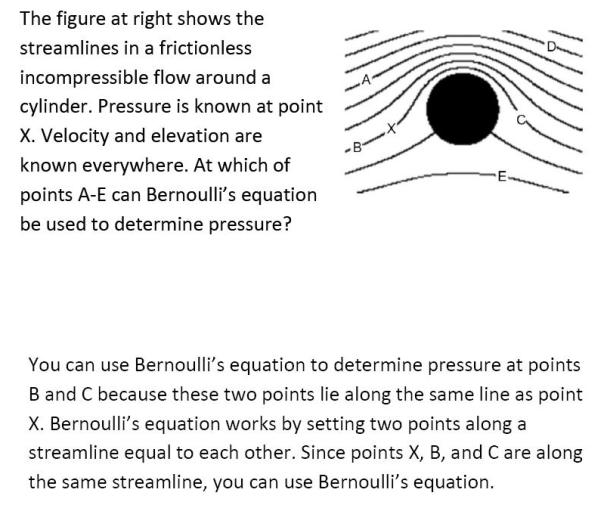fluid mechanics math problems engineering equations fluid mechanics basic mechanics school homework engineering math fluids formulas fluid mechanics problems fluid mechanics problem solutions to fluids problems full solution fluid mechanics engineering fluids problem solution fluid mechanics math problems engineering equations fluid mechanics basic mechanics school homework engineering math fluid mechanics formulas fluid problems fluid mechanics problem solutions to fluid mechanics problems full solution fluid mechanics engineering fluid mechanics problem solution
fluid mechanics math problems engineering equations fluid mechanics basic mechanics school homework engineering math fluids formulas fluid mechanics problems fluid mechanics problem solutions to fluids problems full solution fluid mechanics engineering fluids problem solution fluid mechanics math problems engineering equations fluid mechanics basic mechanics school homework engineering math fluid mechanics formulas fluid problems fluid mechanics problem solutions to fluid mechanics problems full solution fluid mechanics engineering fluid mechanics problem solution
Highalphabet Home Page fluid mechanics solutions fluids math solved Fluid Mechanics Page 1
The figure at right shows the streamlines in a frictionless incompressible flow around a cylinder. Pressure is known at point X. Velocity and elevation are known everywhere. At which of points A-E can Bernoulli's equation be used to determine pressure?The figure at right shows the streamlines in a frictionless incompressible flow around a cylinder. Pressure is known at point X. Velocity and elevation are known everywhere. At which of points A-E can Bernoulli's equation be used to determine pressure?